## 4.1.1 Discretization of the POISSON Equation

To solve partial differential equations numerically, they are usually discretized. For that reason, the domain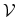where the equations are posed has to be partitioned into a finite number of sub-domains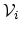, which are usually obtained by a VORONOI tessellation [238,239]. In order to obtain the solution with a desired accuracy, the equation system is approximated in each of these sub-domains by algebraic equations. The unknowns of this system are approximations of the continuous solutions at the discrete grid points in the domain . Several approaches for the discretization of the partial differential equations have been proposed. It has been found to be advantageous to apply the finite boxes discretization scheme for semiconductor device simulation . This method considers the integral form of the equation for each sub-domain, which is the so-called control volumeassociated with the grid point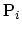. By applying the GAUSS integral theorem, the POISSON equation (4.1) is integrated as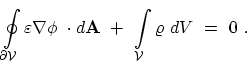(4.3)

Finally, the discretized equation for point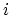with neighbor points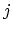can be written implicitly as(4.4)

with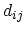is the distance between grid pointand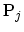,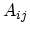as the interface area between the domainsand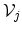, and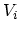as the volume of the domain. For position-dependent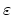, one can use here some average, e.g.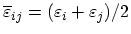. Equation (4.4) can be generally written as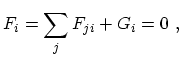(4.5)

whereruns over all neighboring grid points in the same segment,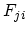is the flux between pointsand, and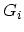is the source term (see Fig. 4.2).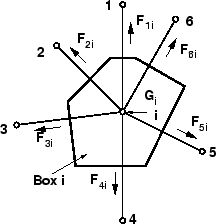Grid points on the boundary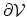are defined as having neighbor grid points in other segments. Thus, for boundary elements (4.5) does not represent the complete control function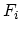, since all fluxes into the contact or the other segment are missing. For that reason, the information for these boxes has to be completed by taking the boundary conditions into account. M. Pourfath: Numerical Study of Quantum Transport in Carbon Nanotube-Based Transistors